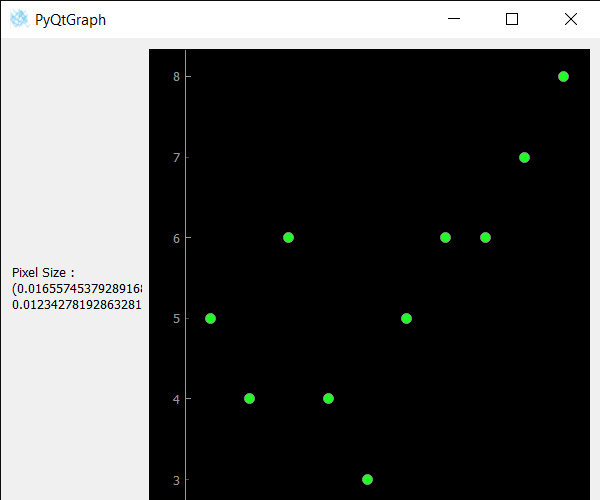PyQtGraph –Getting Pixel Size of Scatter Plot Graph

• Last Updated : 19 Nov, 2021

In this article we will see how we can get pixel size of scatter plot graph of the PyQtGraph module. PyQtGraph is a graphics and user interface library for Python that provides functionality commonly required in designing and science applications. Its primary goals are to provide fast, interactive graphics for displaying data (plots, video, etc.). A scatter plot (aka scatter chart, scatter graph) uses dots to represent values for two different numeric variables. It is a type of plot or mathematical diagram using Cartesian coordinates to display values for typically two variables for a set of data. The position of each dot on the horizontal and vertical axis indicates values for an individual data point. Pixel size is the size of the each pixel of the scatter plot graph.
We can create a plot window and create scatter plot graph on it with the help of commands given below.

# creating a pyqtgraph plot window
plt = pg.plot()

# creating a scatter plot graph of size = 10
scatter = pg.ScatterPlotItem(size=10)

In order to do this we use pixelSize method with the scatter plot graph object
Syntax : scatter.pixelSize()
Argument : It takes no argument
Return : It returns tuple

Below is the implementation

Python3

 # importing Qt widgetsfrom PyQt5.QtWidgets import * # importing systemimport sys # importing numpy as npimport numpy as np # importing pyqtgraph as pgimport pyqtgraph as pgfrom PyQt5.QtGui import *from PyQt5.QtCore import * class Window(QMainWindow):     def __init__(self):        super().__init__()         # setting title        self.setWindowTitle("PyQtGraph")         # setting geometry        self.setGeometry(100, 100, 600, 500)         # icon        icon = QIcon("skin.png")         # setting icon to the window        self.setWindowIcon(icon)         # calling method        self.UiComponents()         # showing all the widgets        self.show()     # method for components    def UiComponents(self):         # creating a widget object        widget = QWidget()         # creating a label        label = QLabel("Geeksforgeeks Scatter Plot")         # making label do word wrap        label.setWordWrap(True)         # creating a plot window        plot = pg.plot()         # number of points        n = 300         # creating a scatter plot item        # of size = 10        # using brush to enlarge the of green color        scatter = pg.ScatterPlotItem(            size=10, brush=pg.mkBrush(30, 255, 35, 255))         # data for x-axis        x_data = [1, 2, 3, 4, 5, 6, 7, 8, 9, 10]         # data for y-axis        y_data = [5, 4, 6, 4, 3, 5, 6, 6, 7, 8]         # setting data to the scatter plot        scatter.setData(x_data, y_data)         # add item to plot window        # adding scatter plot item to the plot window        plot.addItem(scatter)         # Creating a grid layout        layout = QGridLayout()         # minimum width value of the label        label.setMinimumWidth(130)         # setting this layout to the widget        widget.setLayout(layout)         # adding label in the layout        layout.addWidget(label, 1, 0)         # plot window goes on right side, spanning 3 rows        layout.addWidget(plot, 0, 1, 3, 1)         # setting this widget as central widget of the main window        self.setCentralWidget(widget)         # getting pixel size of the scatter plot        value = scatter.pixelSize()         # setting text to the value        label.setText("Pixel Size : " + str(value)) # create pyqt5 appApp = QApplication(sys.argv) # create the instance of our Windowwindow = Window() # start the appsys.exit(App.exec())

Output :Attention geek! Strengthen your foundations with the Python Programming Foundation Course and learn the basics.

To begin with, your interview preparations Enhance your Data Structures concepts with the Python DS Course. And to begin with your Machine Learning Journey, join the Machine Learning - Basic Level Course

My Personal Notes arrow_drop_up